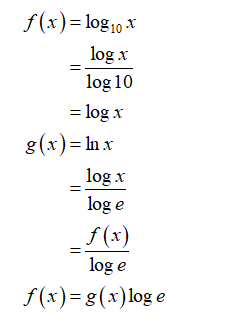# Determine whether f <<g or g<< f (or neither) for the functions f (x) = log10 x and g(x) = ln x.

Question
1 views

Determine whether f <<g or g<< f (or neither) for the functions f (x) = log10 x and g(x) = ln x.

check_circle

Step 1

Convert both lnx and log10x in terms of logx

Step 2

Convert both lnx and log10x in terms of logx...

### Want to see the full answer?

See Solution

#### Want to see this answer and more?

Solutions are written by subject experts who are available 24/7. Questions are typically answered within 1 hour.*

See Solution
*Response times may vary by subject and question.
Tagged in